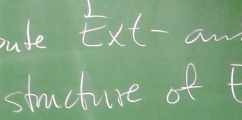BIREP – Representations of finite dimensional algebras at Bielefeld# Seminar on Ringel-Hall Algebras

Time and place: Wednesdays 10-12 in room V3-204

# Ringel-Hall Algebras

In , P. Hall showed how one could construct an algebra by taking as basis the isomorphism classes of finite $p$-groups, and where the multiplication counts all possible extensions of the two groups. This is the classical Hall algebra, or algebra of partitions, so named since the isomorphism classes of finite $p$-groups are in bijection with the set of partitions. As an algebra, this is isomorphic to a polynomial ring on countably many variables, but it has a much richer structure, that of a self-dual Hopf algebra. Nowadays this algebra is often referred to as Macdonald's ring of symmetric functions, and encodes a lot of interesting combinatorics . For example, the Kostka numbers occur as entries of the base-change matrix between the bases of Schur functions and monomial functions. Note that this algebra has a history going back even further; for example it was discussed by Steinitz already in 1901 .

This idea was picked up by Ringel in , who showed that one could attach in a similar way an algebra to any finitary abelian category, the so-called Ringel-Hall algebra; here finitary means that the homomorphism and first extension groups are all finite sets. In particular, this works for the category of finite-dimensional modules over a $k$-algebra whenever $k$ is a finite field, but also for the category of coherent sheaves on projective space, again over a finite field. There is also a natural coalgebra structure, but in general these two structures are not compatible.

In fact, analysing the Ringel-Hall algebra coming from the representations of a quiver, Green showed in  that the multiplication and comultiplication are compatible precisely when the category is hereditary (so the second and higher extension groups all vanish), and in this case we again obtain a self-dual Hopf algebra. Moreover, he proved that a certain natural subalgebra (that generated by the simple objects) is isomorphic, again as a self-dual Hopf algebra, to a quantum group, so the (positive part of the) quantised enveloping algebra of a symmetrisable Kac-Moody Lie algebra . For the case of $p$-groups, or similarly the finite-dimensional modules over the Laurent polynomial ring $k[[t]]$, the Lie algebra is the affinisation (or loop algebra) of $\mathfrak{sl}_2$.

As an application, one can compare the Weyl denominator formula for the quantum group with the natural factorisation of the Ringel-Hall algebra derived from the Krull-Remak-Schmidt Theorem on the module category to ascertain that the dimension vectors of the indecomposable modules are precisely the positive roots of the Kac-Moody Lie algebra . In some sense, this completed the circle, since Kac had already shown that this result for dimension vectors holds , and Ringel was interested in obtaining a deeper structural relationship between the module category and the Kac-Moody Lie algebra suggested by this combinatorial result.

On the other hand, using the natural basis for the Ringel-Hall algebra (more precisely, comparing these bases for the different orientations of the quiver), Lusztig was inspired to construct his canonical basis of the quantum group , which has particularly nice properties with respect to a certain class of modules over the quantum group.

More recently there has been work developing Ringel-Hall algebras for elliptic curves , weighted projective lines , configurations of abelian categories and stacks , as well as for Frobenius categories  and triangulated categories .

A more detailed overview of Ringel-Hall algebras is also available.

# Schedule of Talks

• 13 April: Ringel-Hall algebras (Baolin Xiong)
• 20 April: The algebra of partitions (Andrew Hubery): lecture notes
• 27 April: Green's formula, I (Andre Beineke)
• 04 May: Green's formula, II (Ögmundur Eiriksson)
• 11 May: Quiver representations and quantum Serre relations (Rolf Farnsteiner): lecture notes
• 18 May: The Kronecker quiver (Fajar Yuliawan): Kronecker representations
• 25 May: Coherent sheaves on the projective line (Thomas Cauwbergs)
• 01 June: Quantum groups (Florian Gellert)
• 08 June: Green's main theorem (Philipp Lampe): lecture notes
• 15 June: Exceptional modules and root vectors (Paula Lins)
• 22 June: Quiver Grassmannians (Christopher Voll)

# References

  I. Burban and O. Schiffmann, On the Hall algebra of an elliptic curve, I, Duke Math. J. 161 (2012), 1171-1231  B. Deng and J. Xiao, A new approach to Kac's theorem on representations of valued quivers, Math. Z. 245 (2003), 183-199  M. Gorsky, Semi-derived and derived Hall algebras for stable categories, preprint arXiv:1409.679  J. A. Green, Hall algebras, hereditary algebras and quantum groups, Invent. Math. 120 (1995), 361-377  P. Hall, The algebra of partitions, Proceedings 4th Canadian Mathematical Congress (1959), 147-159  D. Joyce, Configurations in abelian categories. II. Ringel-Hall algebras, Adv. Math. 210 (2007), 635-706  V. G. Kac, Infinite root systems, representations of graphs and invariant theory, Invent. Math. 56 (1980), 57-92  G. Lusztig, Canonical bases arising from quantized enveloping algebras, J. Amer. Math. Soc. 3 (1990), 447-498  G. Lusztig, Introduction to quantum groups, Progress in Math. 110 (Birkhäuser Boston, Boston, MA, 1993)  I. G. Macdonald, Symmetric functions and Hall polynomials. Second edition. With contributions by A. Zelevinsky, Oxford Mathematical Monographs. Oxford Science Publications. (The Clarendon Press, Oxford University Press, New York, 1995)  C. M. Ringel, Hall algebras and quantum groups, Invent. Math. 101 (1990), 583-591  O. Schiffmann, Noncommutative projective curves and quantum loop algebras, Duke Math. J. 121 (2004), 113-168  E. Steinitz, Zur Theorie der Abelschen Gruppen, Jahresberichts der DMV 9 (1901), 80-85  B. Toën, Derived Hall algebras, Duke Math. J. 135 (2006), 587-615